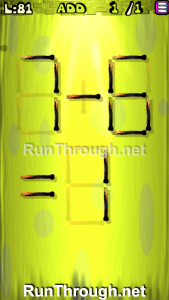# Move the Matches Puzzles Episode 2 Level 81-100 Walkthrough

Walkthrough for all the solutions and answers to the game Move the Matches Puzzles by K-Lab. Get the app on the Google Play Store to play along if you do not already have it.  This is for episode 2, levels 81 through 100.

The format for the walkthrough images have also changed from now on.  Instead of green and red marks where the matches are added and removed, we will be showing the final image of the Matches Puzzle.  This will allow you to see where everything goes, and to know for sure that the puzzle works.

Let us know what you think of this new format!

### Level 81Instructions: Add 1 match to correct the equation

Solution: 7-6=1

### Level 82Instructions: Remove 4 matches to get 6 squares, all equal

### Level 83Instructions: Move 1 match to correct the equation

Solution: 0+1=1

### Level 84Instructions: Add 1 match to correct the equation

Solution: 2+1=3

### Level 85Instructions: Remove 2 matches to correct the equation

Solution: 6-4=2

### Level 86Instructions: Move 2 matches to get 3 triangles

### Level 87Instructions: Move 2 matches to get 2 triangles, all equal

### Level 88Instructions: Add 1 match to correct the equation

Solution: 9-4=5

### Level 89Instructions: Move 2 matches to get 2 triangles

###Instructions: Move 2 matches to correct the equation

Solution: 9-6=3

###Instructions: Move 1 match to correct the equation

Solution: 6/6=1

###Instructions: Remove 2 matches to correct the equation

Solution: 2+3=5

###Instructions: Remove 3 matches to correct the equation

Solution: 4+9=13

###Instructions: Remove 4 matches to get 5 triangles, all equal

###Instructions: Move 1 match to correct the equation

Solution: 8×5=40

###Instructions: Remove 7 matches to get 2 triangles

###Instructions: Move 1 match to correct the equation

Solution:  9+9=18

###Instructions: Remove 2 matches to correct the equation

Solution: 8/4=2

###Instructions: Add 2 matches to get 2 triangles

### Level 100Instructions: Remove 2 matches to get 6 triangles, all equal